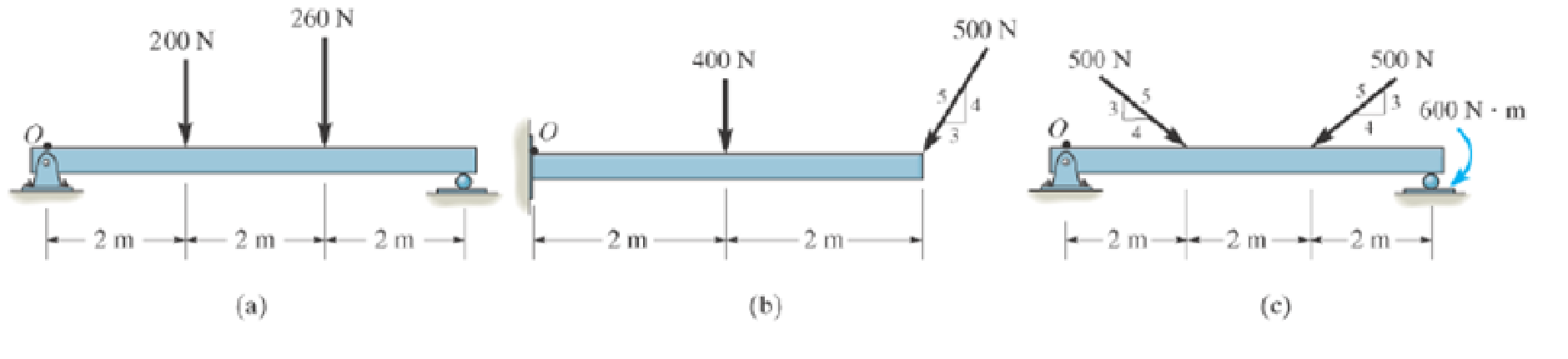#### Concept explainers#### Videos

Textbook QuestionChapter 4.8, Problem 6PP

P4–6. In each case, determine the x and y components of the resultant force and specify the distance where this force acts from point O.10:53
Students have asked these similar questions
Find the sum of the moments of the two forces O about the point F and P
The cable AB prevents bar OA from rotating clockwise about the pivot O. If the cable tension is 850 N, determine the n- and t-components of this force acting on point A of the bar.
If F=1kN,the magnitude of the vertical reaction force developed at the point B?